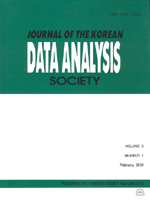상세검색
최근 검색어 전체 삭제
다국어입력
즐겨찾기0KCI등재 학술저널

# Aggregated Ratio Imputation for e-Business Survey

• 등재여부 : KCI등재
• 2010.04
• 663 - 670 (8 pages)

Business surveys typically collect responses on a large number of items for each unit sampled. However, a survey will rarely achieve a one hundred percent response rate on all the items. Some or all of the responses may be missing for a sample unit. Korea Electronics Association(KEA) collect monthly survey data and they fail to get a response on the desired data items. One approach to dealing with this problem was touched in this study, so that the whole population is represented by just the respondents. As usual KEA survey data have a strong correlation among the monthly responses and hence we applied aggregated ratio imputation to this data set. Generally, an aggregate ratio impute is calculated by applying a ratio calculated from similarly behaved units to some existing data values for the unit requiring imputation. The most common example is to apply a growth factor to the value recorded by the unit on the variable of interest on the previous period, where the growth factor is estimated from the response of units with similar characteristics. Applying this approach for KEA data set we get a good performance and final results are shown in the conclusion part.

1. Introduction

2. Imputation

3. Conclusion

References